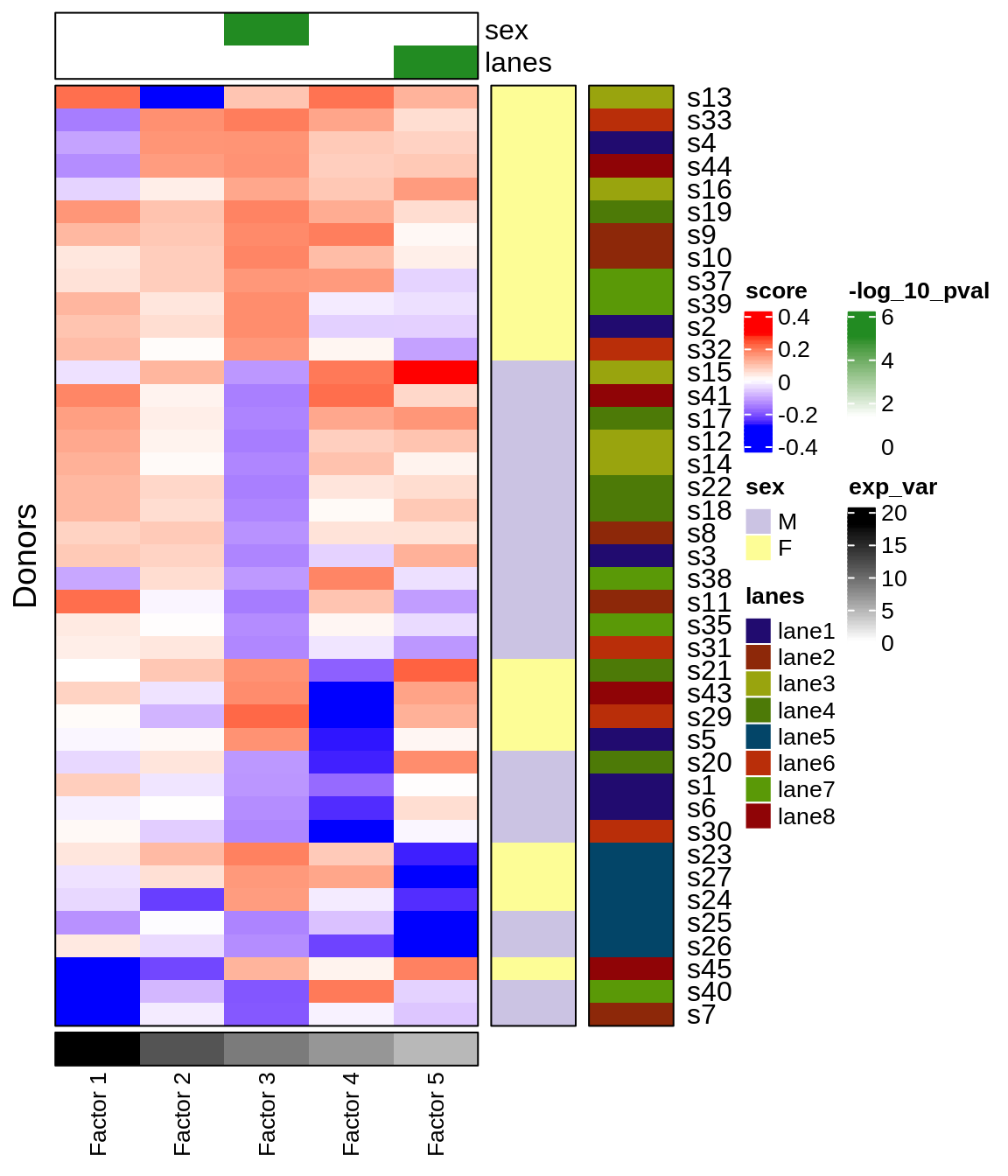You can download the data used in this tutorial with the links listed below. It is a single-cell RNA-seq dataset of peripheral blood mononuclear cells from 45 healthy donors from a study by van der Wijst et al. (2018).

1. scITD requires gene x cell barcode UMI matrix. This object should be of class ‘dgCMatrix’ or ‘matrix’: http://pklab.med.harvard.edu/jonathan/data/pbmc_counts_v2.rds

2. scITD also requires metadata corresponding to each cell. At a minimum, the meta data must include columns labeled ‘donors’ and ‘ctypes’, but it may include other variables of interest. This object should be of class ‘data.frame’: http://pklab.med.harvard.edu/jonathan/data/pbmc_meta_v2.rds

3. If the genes are labeled with symbols other than gene symbols, it can be helpful to provide the data for converting gene names. The following object is of class ‘data.frame’: http://pklab.med.harvard.edu/jonathan/data/genes.rds

The code used to produce/clean these objects can be found here: http://pklab.med.harvard.edu/jonathan/data/PBMC_preprocessing.R

library(scITD)

# counts matrix

# meta data matrix

# ensembl to gene name conversions
feature.names <- readRDS('/home/jmitchel/data/van_der_wijst/genes.rds')

Next, we need to set up a project container. This is done in two steps:
1. Set project parameters, specifying the cell types to include
2. Create an object to hold the data as well as results and other information

# set up project parameters
param_list <- initialize_params(ctypes_use = c("CD4+ T", "CD8+ T", "cMonocyte", "CD56(dim) NK", "B"),
ncores = 30, rand_seed = 10)

# create project container
pbmc_container <- make_new_container(count_data=pbmc_counts,
meta_data=pbmc_meta,
gn_convert = feature.names,
params=param_list,
label_donor_sex = TRUE)

Since our meta data did not have information regarding donor sex, we set label_donor_sex = TRUE to extract this information from the expression of sex-specific genes. This new information is automatically added to our metadata. While having this information is not absolutely necessary, it can be useful later on to see if we identify a factor that is associated with sex.

Next, we will form our data into a tensor with dimensions of donors x genes x cell-types. The parameters norm_method and scale_factor are used for normalizing the pseudobulked data. The parameters vargenes_method and vargenes_thresh are used for identifying overdispersed genes. Lastly, scale_var and var_scale_power are used for scaling the variance of each gene in each cell type slice of the final tensor.

The settings shown here will work well for most data, though it is worth playing with the var_scale_power if a sex-associated factor is not apparent. Typically a var_scale_power value between 0.5-2 yields good results. See the documentation for further details with all parameters.

# form the tensor from the data
pbmc_container <- form_tensor(pbmc_container, donor_min_cells=5,
norm_method='trim', scale_factor=10000,
vargenes_method='norm_var_pvals', vargenes_thresh=.1,
scale_var = TRUE, var_scale_power = 2)
#>  "parsing data matrix by cell/tissue type..."
#>  "cleaning data..."
#>  "Keeping 41 donors. All donors have at least 5 cells in each cell type included."
#>  "collapsing count matrices from cells to donors (aka pseudobulk operation)..."
#>  "normalizing data..."
#>  "calculating gene overdispersion factors..."
#>  "selecting highly variable genes from each cell type..."
#>  "scaling variance..."
#>  "forming tensor..."
#>  "Complete!"

After running this function, one should check the number of overdispersed genes identified to make sure the tensor has a decent number of genes before running the decomposition. This can be checked by running the following line of code:

# number of genes included in the tensor
print(length(pbmc_container[["all_vargenes"]]))
#>  741

To increase the number of genes included, simply rerun form_tensor() with a higher vargenes_thresh value.

We are now ready to run the Tucker decomposition and analyze the interesting biological variation present in our dataset! We specify rotation_type='hybrid' to indicate that we want to optize the factor loadings using a hybrid rotation method. This improves interpretability of the factors by making the gene patterns more modular/independent from one another. By setting ranks=c(5,10), we indicate that we want to extract 5 multicellular processes that vary across donors using 10 gene sets. To determine an appropriate ranks setting, there are a helpful tips we suggest at the bottom of this tutorial.

# run the tensor decomposition
pbmc_container <- run_tucker_ica(pbmc_container, ranks=c(5,10),
tucker_type = 'regular', rotation_type = 'hybrid')

Next, we will plot the donor scores matrix that tells us the level to which each process/factor is present in each donor. We also show some associations between the donor scores and two metadata variables, sex and lanes.

# get donor scores-metadata associations
pbmc_container <- get_meta_associations(pbmc_container, vars_test=c('sex','lanes'), stat_use='pval')

# plot donor scores
pbmc_container <- plot_donor_matrix(pbmc_container, meta_vars=c('sex','lanes'),
show_donor_ids = TRUE,

# show the donor scores heatmap
pbmc_container$plots$donor_matrixWe can see that factor 3 is significantly associated with donor sex and factor 5 is significantly associated with the 10x lane batch.

Next, it will be useful to determine which genes from each cell type are significantly associated with each factor. This is done as follows:

# get significant genes
pbmc_container <- get_lm_pvals(pbmc_container)

Now let’s look at the corresponding loadings matrices for each factor reduced to only the significant genes.

# generate the loadings plots
pbmc_container <- get_all_lds_factor_plots(pbmc_container,
use_sig_only=TRUE,
nonsig_to_zero=TRUE,
sig_thresh=.02,
display_genes=FALSE,
gene_callouts = TRUE,
callout_n_gene_per_ctype=3,
show_var_explained = TRUE)

# arrange the plots into a figure and show the figure
myfig